# Boolean Algebra 1 M AL Towaileb Boolean Functions

• Slides: 22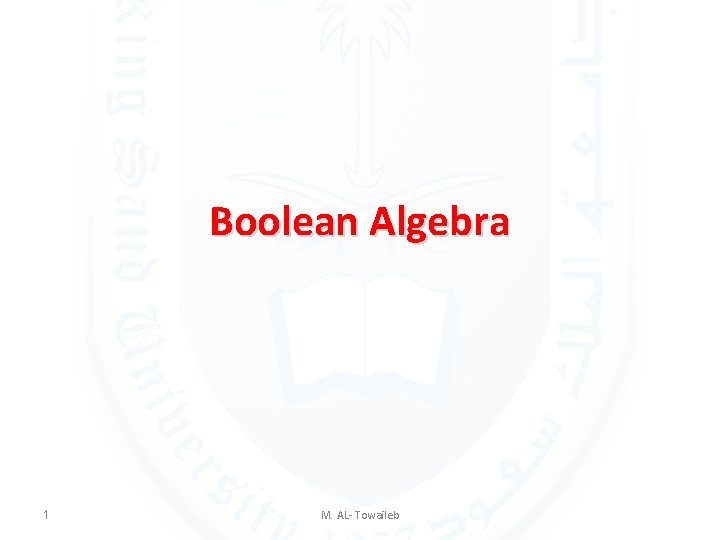Boolean Algebra 1 M. AL- Towaileb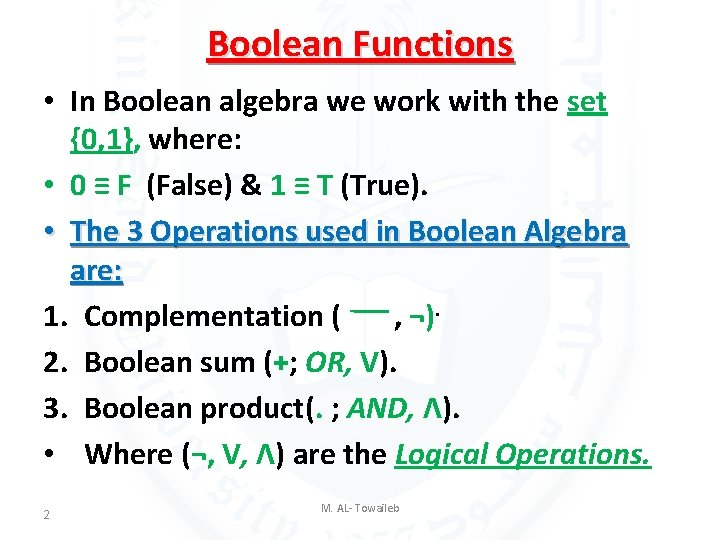Boolean Functions • In Boolean algebra we work with the set {0, 1}, where: • 0 ≡ F (False) & 1 ≡ T (True). • The 3 Operations used in Boolean Algebra are: 1. Complementation ( - ــــ , ¬). 2. Boolean sum (+; OR, V). 3. Boolean product(. ; AND, Λ). • Where (¬, V, Λ) are the Logical Operations. 2 M. AL- Towaileb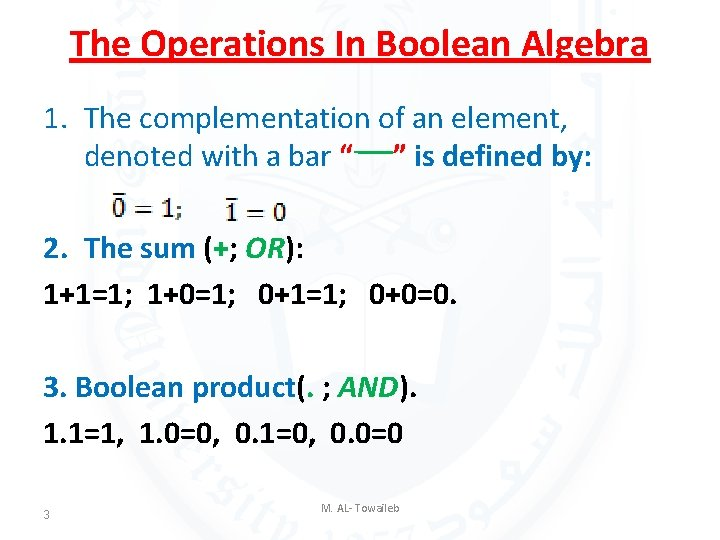The Operations In Boolean Algebra 1. The complementation of an element, denoted with a bar “- ”ــــ is defined by: 2. The sum (+; OR): 1+1=1; 1+0=1; 0+1=1; 0+0=0. 3. Boolean product(. ; AND). 1. 1=1, 1. 0=0, 0. 1=0, 0. 0=0 3 M. AL- Towaileb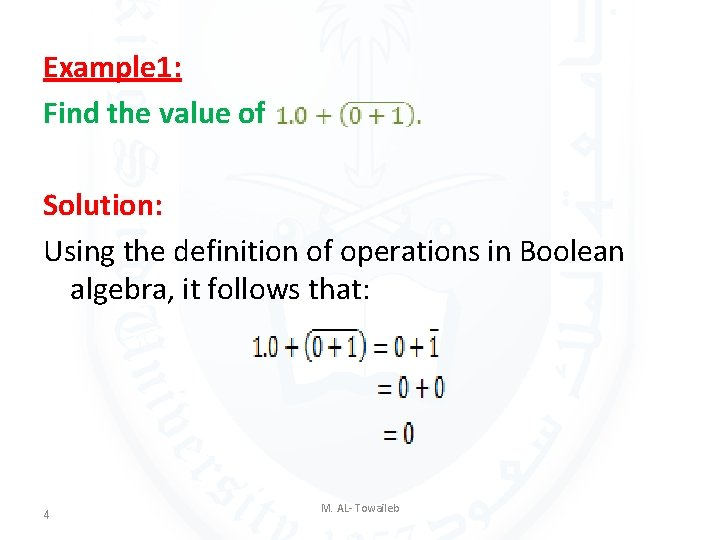Example 1: Find the value of Solution: Using the definition of operations in Boolean algebra, it follows that: 4 M. AL- Towaileb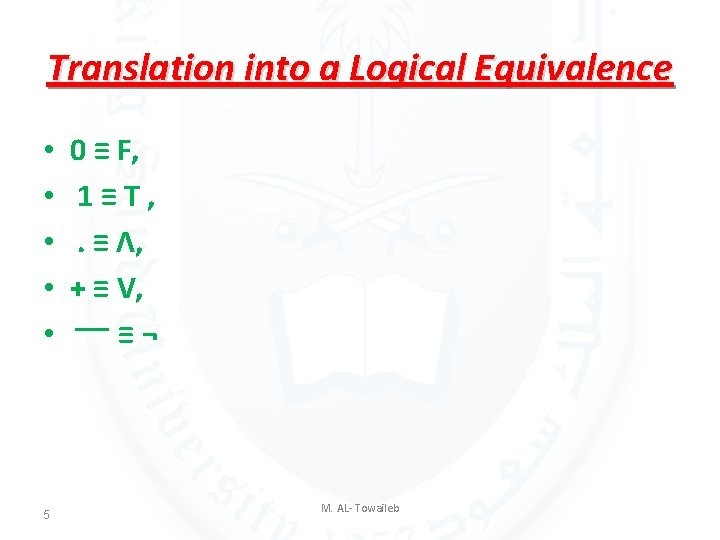Translation into a Logical Equivalence • • • 5 0 ≡ F, 1≡T, . ≡ Λ, + ≡ V, ¬ ≡ ــــ M. AL- Towaileb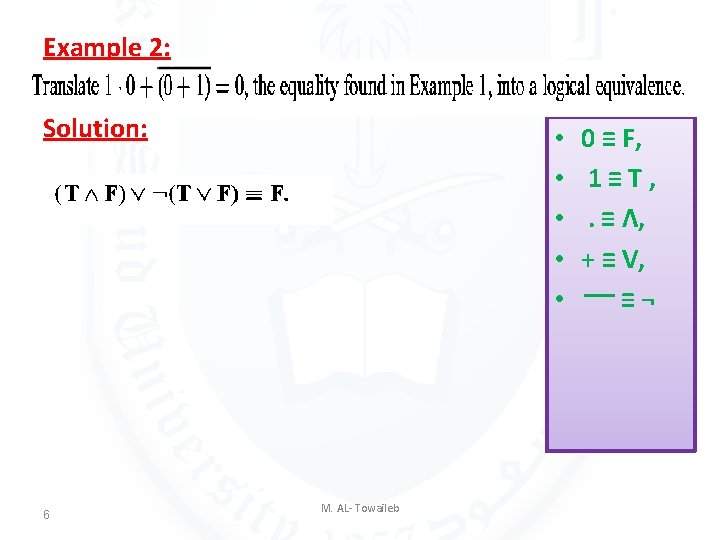Example 2: Solution: 6 • • • M. AL- Towaileb 0 ≡ F, 1≡T, . ≡ Λ, + ≡ V, ¬ ≡ ــــExample 3: • • • Solution: 7 M. AL- Towaileb 0 ≡ F, 1≡T, . ≡ Λ, + ≡ V, ¬ ≡ ــــBoolean Expressions and Boolean Functions Let B= {0, 1}. Then Bn={(x 1, x 2 , • • • , xn)/xiϵB for 1 ≤ i ≤n} is the set of all possible n -tuples of Os and 1 s. The variable x is called a Boolean variable if it assumes values only from B , that is, if its only possible values are 0 and 1. A function from Bn to B is called a Boolean function of degree n. 8 M. AL- Towaileb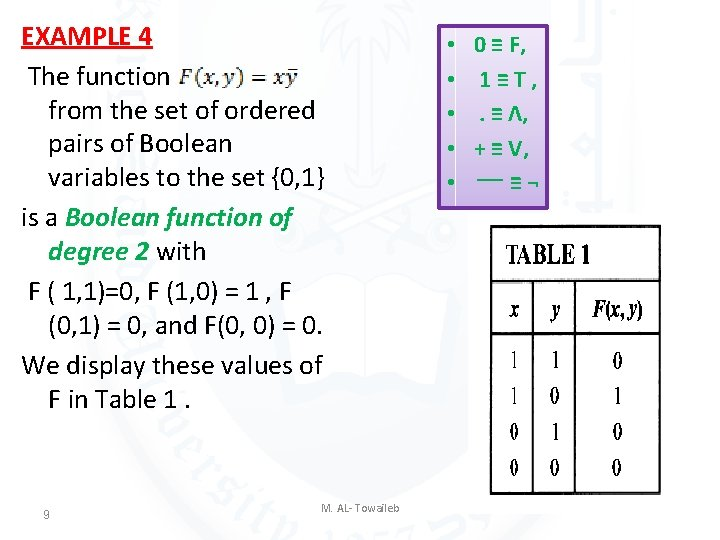EXAMPLE 4 The function from the set of ordered pairs of Boolean variables to the set {0, 1} is a Boolean function of degree 2 with F ( 1, 1)=0, F (1, 0) = 1 , F (0, 1) = 0, and F(0, 0) = 0. We display these values of F in Table 1. 9 M. AL- Towaileb • • • 0 ≡ F, 1≡T, . ≡ Λ, + ≡ V, ¬ ≡ ــــ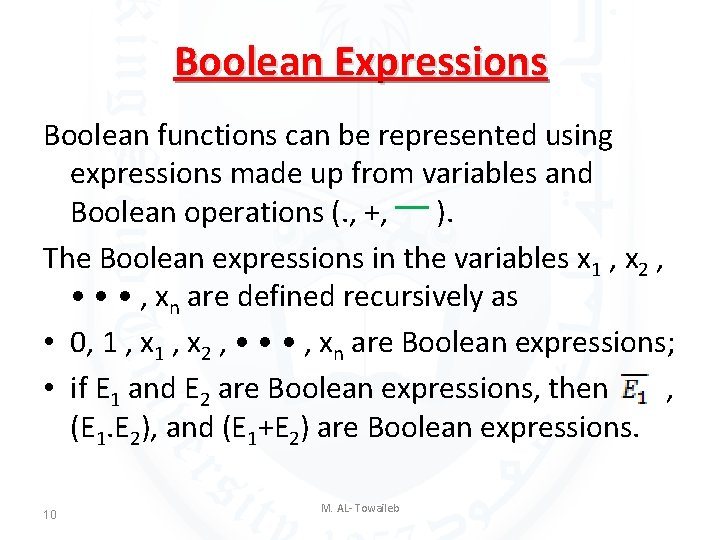Boolean Expressions Boolean functions can be represented using expressions made up from variables and Boolean operations (. , +, ) ــــ. The Boolean expressions in the variables x 1 , x 2 , • • • , xn are defined recursively as • 0, 1 , x 2 , • • • , xn are Boolean expressions; • if E 1 and E 2 are Boolean expressions, then , (E 1. E 2), and (E 1+E 2) are Boolean expressions. 10 M. AL- TowailebEXAMPLE 5 Find the values of the Boolean function represented by Solution: The values of this function are displayed in Table 2. 11 M. AL- Towaileb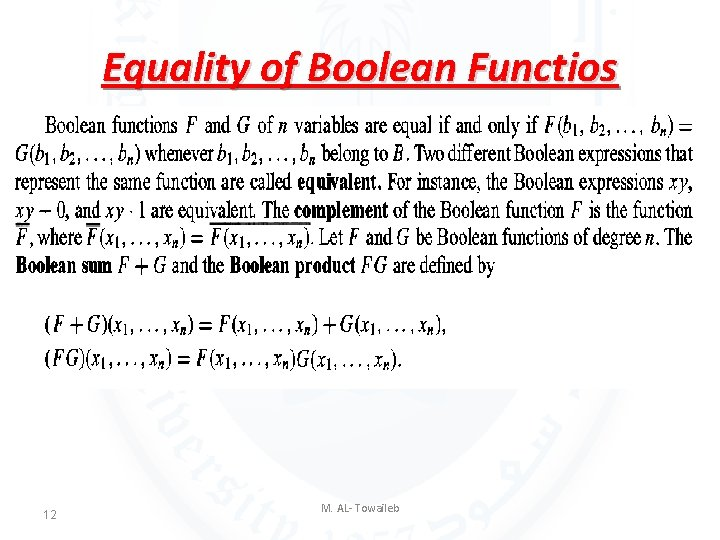Equality of Boolean Functios 12 M. AL- Towaileb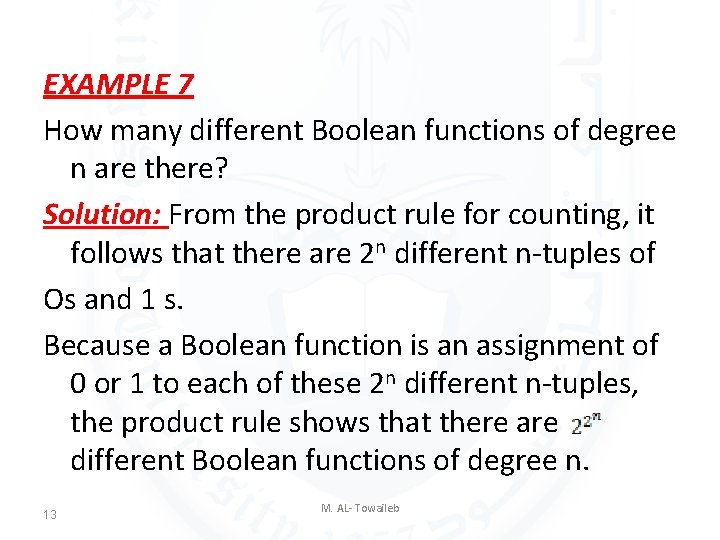EXAMPLE 7 How many different Boolean functions of degree n are there? Solution: From the product rule for counting, it follows that there are 2 n different n-tuples of Os and 1 s. Because a Boolean function is an assignment of 0 or 1 to each of these 2 n different n-tuples, the product rule shows that there are different Boolean functions of degree n. 13 M. AL- Towaileb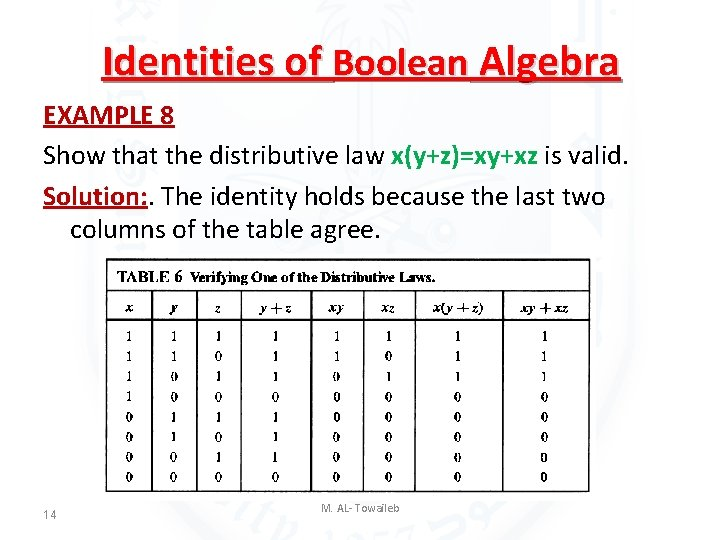Identities of Boolean Algebra EXAMPLE 8 Show that the distributive law x(y+z)=xy+xz is valid. Solution: . The identity holds because the last two columns of the table agree. 14 M. AL- Towaileb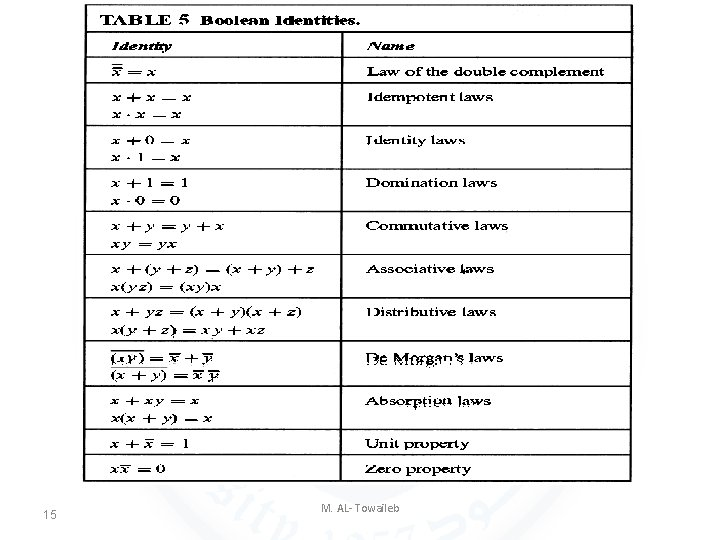15 M. AL- Towaileb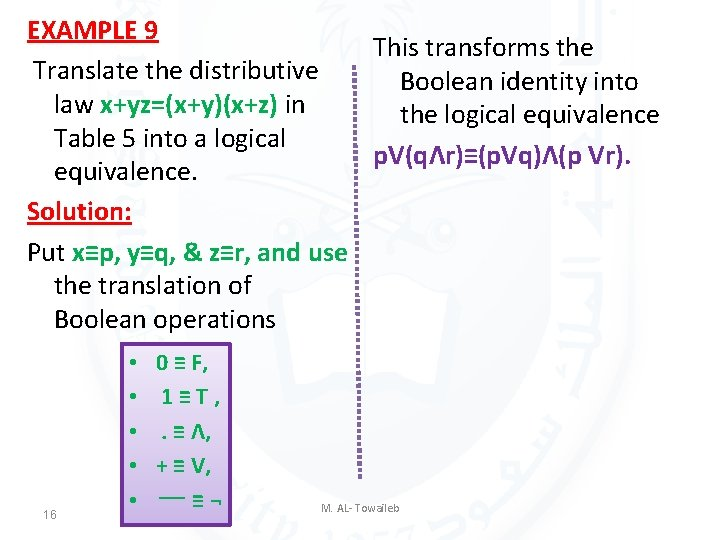EXAMPLE 9 This transforms the Translate the distributive Boolean identity into law x+yz=(x+y)(x+z) in the logical equivalence Table 5 into a logical p. V(qΛr)≡(p. Vq)Λ(p Vr). equivalence. Solution: Put x≡p, y≡q, & z≡r, and use the translation of Boolean operations 16 • • • 0 ≡ F, 1≡T, . ≡ Λ, + ≡ V, ¬ ≡ ــــ M. AL- Towaileb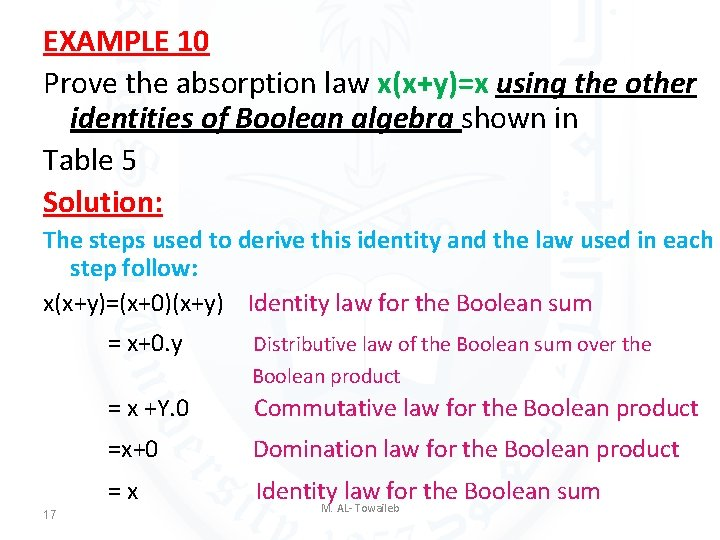EXAMPLE 10 Prove the absorption law x(x+y)=x using the other identities of Boolean algebra shown in Table 5 Solution: The steps used to derive this identity and the law used in each step follow: x(x+y)=(x+0)(x+y) Identity law for the Boolean sum 17 = x+0. y Distributive law of the Boolean sum over the Boolean product = x +Y. 0 Commutative law for the Boolean product =x+0 Domination law for the Boolean product =x Identity law for the Boolean sum M. AL- Towaileb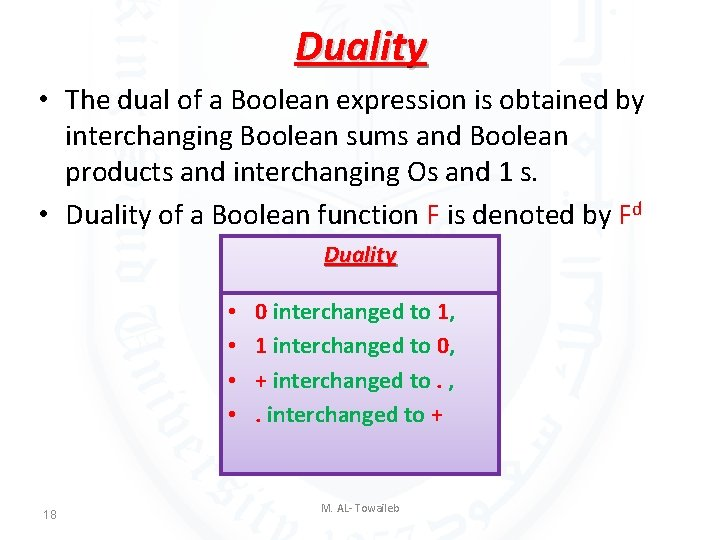Duality • The dual of a Boolean expression is obtained by interchanging Boolean sums and Boolean products and interchanging Os and 1 s. • Duality of a Boolean function F is denoted by Fd Duality • • 18 0 interchanged to 1, 1 interchanged to 0, + interchanged to. , . interchanged to + M. AL- Towaileb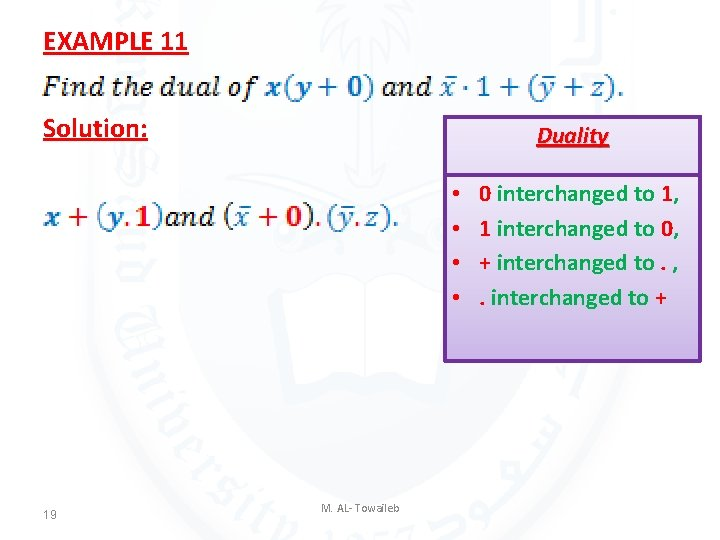EXAMPLE 11 Solution: Duality • • 19 M. AL- Towaileb 0 interchanged to 1, 1 interchanged to 0, + interchanged to. , . interchanged to +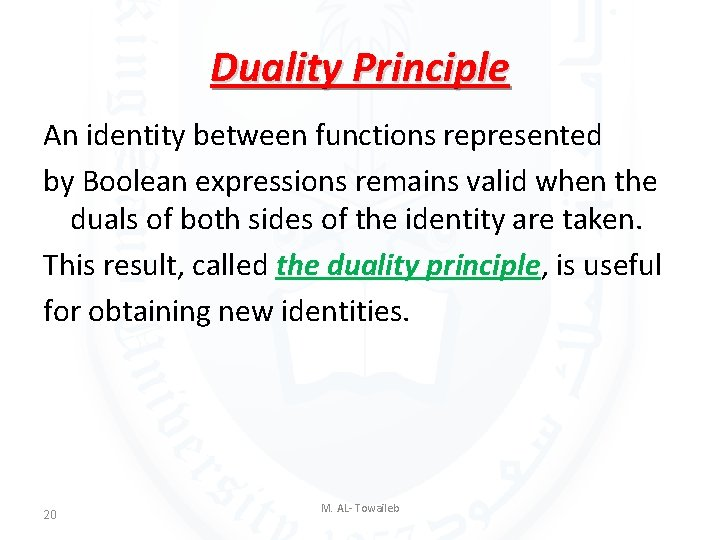Duality Principle An identity between functions represented by Boolean expressions remains valid when the duals of both sides of the identity are taken. This result, called the duality principle, is useful for obtaining new identities. 20 M. AL- Towaileb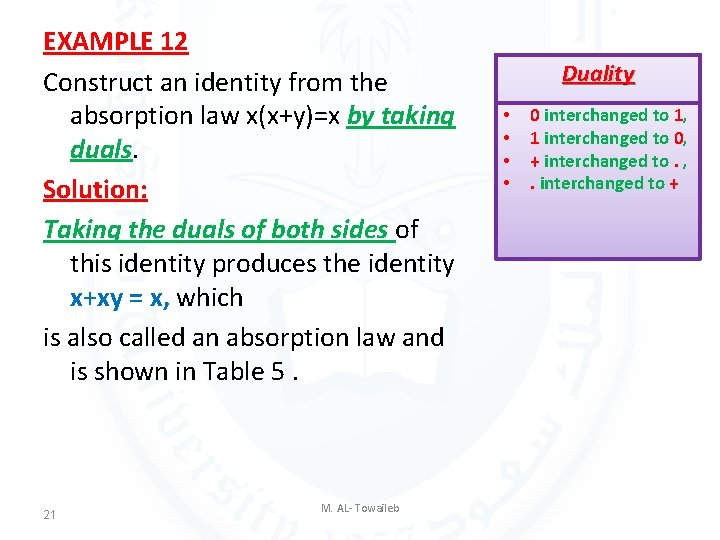EXAMPLE 12 Construct an identity from the absorption law x(x+y)=x by taking duals. Solution: Taking the duals of both sides of this identity produces the identity x+xy = x, which is also called an absorption law and is shown in Table 5. 21 M. AL- Towaileb Duality • • 0 interchanged to 1, 1 interchanged to 0, + interchanged to. , . interchanged to +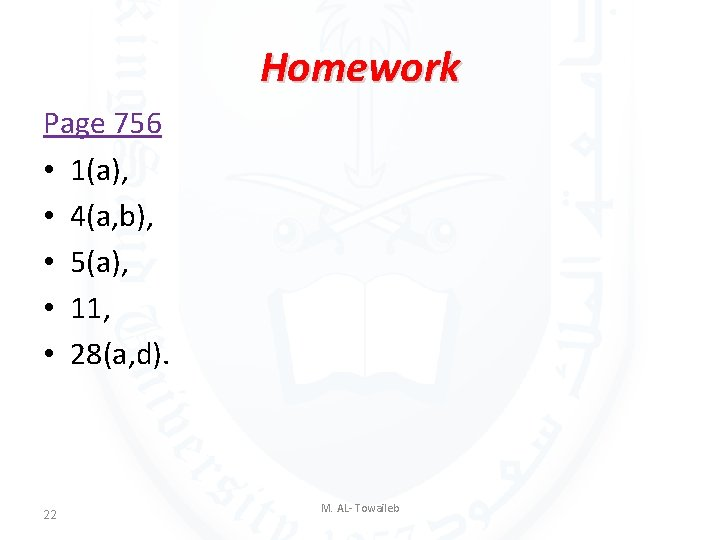Homework Page 756 • 1(a), • 4(a, b), • 5(a), • 11, • 28(a, d). 22 M. AL- Towaileb